### Order new ME502 Overview of Digital Communication Assignment & Solution at nominal price only from Expertsminds.com!

Home   Course   ME502 Overview of Digital Communication Assignment Help
Previous << || >> Next

ME502 Overview of Digital Communication Assignment Help

Question 1: Amplitude Modulation (AM)

Consider the message or modulating signal m(t)=Amcos(2πf0t) with frequency f0=60 Hz, amplitude Am=4V and the carrier signal c(t)= Accos(2πfct + φ) with voltage amplitude Ac=1V, frequency fc=800 MHz and phase φ=π/4. We want to compare suppressed carrier AM (SC-AM) and full-carrier AM (FC- AM) and their variants.

DSB-SC AM signal: u(t)=m(t)c(t)

DSB-FC AM signal: v(t)=[1 + m(t)/Ac]*c(t)

(a) Using Matlab, sketch the waveforms of m(t), u(t)=m(t)c(t) and v(t)=[1 + m(t)/Ac]*c(t) in one figure. Explain what you observe from the figures.

(b) Using Matlab sketch the spectra of m(t), u(t)=m(t)c(t) and v(t)=[1 + m(t)/Ac]*c(t) in one figure.

Question 2: Huffman coding

Consider a source with the seven symbols in its alphabet s = {'s0' 's1' 's2' 's3' 's4' 's5' 's6'} which has the probabilities p = [0.18 0.21 0.2 0.26 0.03 0.05 0.07].

(a) Write a MATLAB function ‘entropy’ to compute the entropy of the source.

(b) Use your function ‘entropy’ to find the entropy of the given source.

(c) Write a MATLAB program to generate a Huffman code for the source.

Work together with Expertsminds tutor to achieve success in ME502 Overview of Digital Communication Assignment Help service!

Introduction

The assignment mainly focuses onto the understanding of resource allocation techniques that are currently deployed for uplink in modern digital architecture, mainly the wireless communication system. This however is mainly achieved through the means of wireless communication methods. The assignment would be focused onto intricate learning of resource allocation techniques which are used into the modern digital architecture, however to understand so the studies of individual wireless communication techniques would be paid close attention.

Scope of the research

The main scope of the research is to study the multi user allocation technique thus the first hurdle of understanding the allocation technique is to be tackled. In simpler terms multi user resource allocation is basically the process through which data or resource is allocated to multiple users in a connected system. However, when the scenario is for a single user the problem is less complex than that of the multi user allocation system. Numerous techniques have been thought about and have been applied for the achieving a simpler yet effective process of uplink into a wireless communication, and the research paper is thoroughly based onto these analyses. However, the allocation of any resource is precisely dependent onto the spectrum and the bandwidth allowed to a wireless communication. In the case of allocation of resource in a multiuser system, the desired allocation solution or size is attained by multiple decompositions. The load to the network is mainly denoted by the number of participants onto the system or the network coverage system. For the desired efficiency of the network system, a higher or a finitely equivalent spectral width has to be achieved. Through the findings of the research, it was observed that technologies which had been in use till date had many a flaws, thus, which was overcome with the help of a newer and a better technological replacement. The current technological advancements working in a unified vision to provide all a better uplink into the wireless system.

Issues in resource allocation

During the past few decades the usability and the adaption to long-range communication had faced a tremendous growth, thus with time came numerous limitations and with such limitations in place, the technological advancements had to be catalysed to come up with never seen before technical advancements. The growth in use of wireless communication gave rise to numerous devises such as cellular devices which helped into downlink and uplink of information. Here, downlink specifically demotes the ability of a node or a device to be able to receive a network signal from the signal distributor, such as satellite; the uplink in the same manner is the ability to upload a send a signal from the participant to the signal distributor or the satellite. From a network point of view more the recipients the higher potential of a failed or an efficient communication network. However, to battle the situation there had been multiple inclusions such as from the Jurassic technology of UMTS(Universal Mobile Telecommunication Service) to the new age technology of LTE Advanced (Advanced Long Term Evolution). The new technology which has been in talk for quite some time now, 5G or fifth generation networking system, is basically the use of short wavelength signals with a higher frequency thus allowing into the use of least compromise in signal strength.

Critical review

The uplink in multi user wireless network is associated to numerous schemes, thus understanding and comparing the schemes are an important part of gaining the knowledge. The basic schemes that are used for the application of uplink into a multi user or multi participant wireless communication grid are the entities of spectral algorithms, matrix based algorithms, pattern based algorithms, search space spectrums and finally to implement such scenarios comes in the use of scheduling strategies. The association of uplink utilising LTE comprises of many aspects such as Heterogeneous Delay which is overcome with the help of a detailed study in Little's Law and studying power and data packet criteria delay. However, LTE Uplink could also be studied using the schemes such as Adaptive Power-efficient scheduler thus, it mainly comprises delay analysis of the adaptive system model of the wireless network grid and utilizing the MATP design proposed for the grid, however, Heuristic algorithm has been supported to be one of the best algorithms to gain understanding to such problem statements.

Literature review of Wireless technologies

There are quite a few available methods of wireless communication, with their distinct flavours of pros and cons. However, over the research paper the consideration would be limited to most widely used ones. The study would let in understanding the wireless system in an intricate manner; the wireless technologies in consideration are as follows:

LTE-Advanced - is also known as the 5G network. It basically serves as the next biggest step into wireless communication. The network basically uses a massive bandwidth with repeaters in proposed intervals thus not letting the depletion of signal strength. However, this also cause the possibility of health depletion thus causing pure scepticism towards the upcoming technology.

LTE - is also broadly known as 4G and is widely used into the current communication lines. The networking system uses a wide bandwidth and uses GSM/EDGE based data terminal technologies, thus resulting into a hybrid technology. However, the result is a much stable and a faster uplink; it purely is due to the combination of different radio signals together to form a stronger network.

Mobile WIMAX - which is also known as Worldwide Interoperability for Microwave Access is basically the standardization of mobile or cellular connectivity all over the world; this was the basic world transforming technology which introduced wireless data access instantaneously, in technical terms it is also known as the 802.16e protocol.

CDMA2000 - which is also widely known as the 3G network or the 3rd generation of wireless networking. The CDMA2000 technology is basically a part of the 3G networking system and was basically considered as the epitome of wireless networking advancements in the last decade. When introduced it was the only system that could allow high frequency data transfer over the entity of cellular devices however the bandwidth being limited as per current standards, the downlink was preferred over the explicitness of uplink thus resulting in a slower uplink, which turned out to be a huge drawback for the technology.

WCDMA - is also known as Wideband Code Division Multiple Access and is the earliest stage of 3G networking models into the physical world. It used to be a widely used wireless network system that could support high speed data transferring protocols, for its time of origin, other than the improved entities of long distance cellular voice transmitting, MMS and messages.

UMTS - is basically considered as the mother of all high speed data transferring wireless network. Universal Mobile Telecommunications System or UMTS is the main pioneer of 3G systems all over the world and purely the existence of data transferring wireless systems. The communication grid could in theory support a data transferring speed of 2Mbps (Megabits per second) however, the system couldn't beer too much nodes or load.

Recent developments

The world around us is constantly changing and so is its needs, however, the need of increased speed from the previous generation of technology remains unchanged. The widely used 4G or the 4th generation of network supports a speed of 300Mbps (Megabits per second), theoretically. However, such a speed remains to be compromised due to the finite number of satellites that are accessible by the government or the telecommunication companies. Thus, the introduction of a newer and a better technology comes into the question, 5th Generation wireless network. The 5G network reportedly is able to tap into a speed 100time faster than that of the previous generation. As per the data, 5th generation wireless network supports a speed of somewhere around 100Gbps (Gigabits per second). The speed being of a higher range, the spectrum allowed and the bandwidth allowed is much higher than the other thus this allows the scope of having a higher uplink speed, in general terms or rather in the generic theories the speed comes to somewhere around 33Gbps.
Wireless technologies may be of several kinds and radio signals are the most widely used once, however, the technology changes with time and similarly this happened when the technology of Wi-Fi was introduced; the technology is heavily used worldwide for data transferring with a higher range of uplink however, this may be compromised due to several materialistic interferences. To battle such a significant scenario, IBM is currently working towards a wireless communication method of Li-Fi which remains similar to the idea of Wi-Fi but instead of electronic radio frequency spectrum, the revolutionary technology uses light as the main spectrum of transfer thus the speed of data transfer remains to be equivalent to the speed of light. However, there are certain limitations thus is currently not feasible into current infrastructure.

Question 1: Amplitude Modulation (AM)
a.
Ac=input('enter carrier signal amplitude');
Am=input('enter message signal amplitude');
fc=input('enter carrier frequency');
fm=input('enter message frequency');% fm<fc
t=0.1;
m=4*cos(2*pi*fm*t);
c=cos(2*pi*fc*t+0.78);
t1=linspace(0,t,1000);
y1=sin(2*pi*fm*t1); % message signal
y2=sin(2*pi*fc*t1); % carrier signal
eq=(1+m.*y1).*(Ac.*y2);
subplot(311);
plot(t1,y1);
xlabel('Time');
ylabel('Amplitude');
title('Message signal');
subplot(312);
plot(t1,y2);
xlabel('Time');
ylabel('Amplitude');
title('Carrier signal');
subplot(313);
plot(t1,eq);
plot(t1,eq,'r');
xlabel('Time');
ylabel('Amplitude');
title('Modulated signal');
grid on;

The program was determined considering the message signal equation as 4*cos(2*pi*fm*t) and the carrier signal equation as cos(2*pi*fc*t+0.78). This will help in developing the amplitude modulated signal in forms of sinusoidal wave forms. The final output signal for the equations are given below,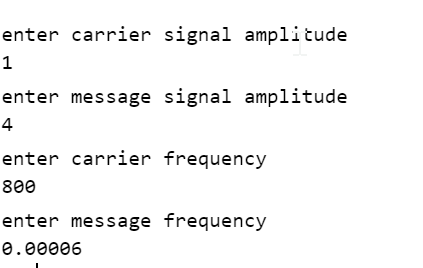The carrier signal amplitude was entered the value of 1 since the values was given to be 1V.The message signal amplitude was entered the value of 4 since the values was given to be 4V. The carrier frequency was entered as 800 as the values was given to be the value of 800 MHz. The message cariier frequency was entered as 0.00006 as the value was given to be 60Hz. The message, carrier, and modulated signal was attained in the Matlab. After entering the values, the following diagrams were enabled,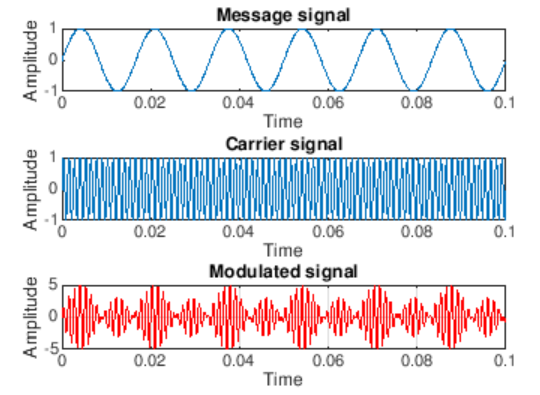b.

The overlapping of the two signal waves were observed and their frequency values composite for determining the peak voltage amplitude. The spectra of the modulated amplitude is developed using the specific and aligned management of the facilities. The spectra of the modulated amplitude is shown below,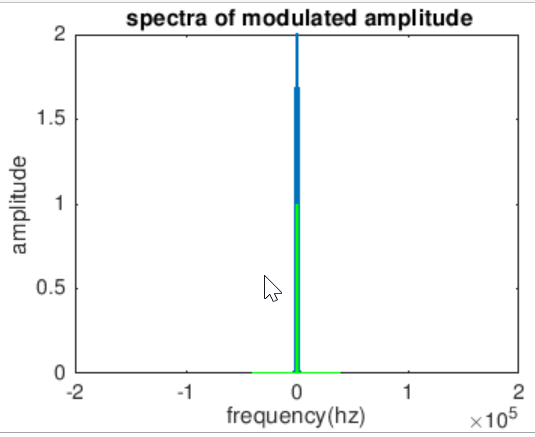Question 2: Huffman coding

The respective probabilities were entered and the diagram was seen as the final output,
a.
H=0;
Z=0;
for(k=1:n)
H=H+(p(k)*log2(1/p(k)));
end
fprintf(1,'Entropy is %f bits',H);
N=H/avglen;
fprintf('\n Efficiency is:%f',N);
for(r=1:n)
l(r)=length(t{r});
end
m=max(l)
s=min(l)
v=m-s;
fprintf('the variance is:%d',v);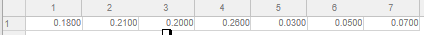c.
clc;
p=input('Enter the probabilities:');
n=length(p);
symbols=[1:n];
[dict,avglen]=huffmandict(symbols,p);
temp=dict;
t=dict(:,2);
for i=1:length(temp)
temp{i,2}=num2str(temp{i,2});
end
disp('The huffman code dict:');
disp(temp)
fprintf('Enter the symbols between 1 to %d in[]',n);
sym=input(':')
encod=huffmanenco(sym,dict);
disp('The encoded output:');
disp(encod);
bits=input('Enter the bit stream in[];');
decod=huffmandeco(bits,dict);
disp('The symbols are:');
disp(decod);

H=0;
Z=0;
for(k=1:n)
H=H+(p(k)*log2(1/p(k)));
end
fprintf(1,'Entropy is %f bits',H);
N=H/avglen;
fprintf('\n Efficiency is:%f',N);
for(r=1:n)
l(r)=length(t{r});
end
m=max(l)
s=min(l)
v=m-s;
fprintf('the variance is:%d',v);
The entering of the values as shown below,
[0.18 0.21 0.2 0.26 0.03 0.05 0.07]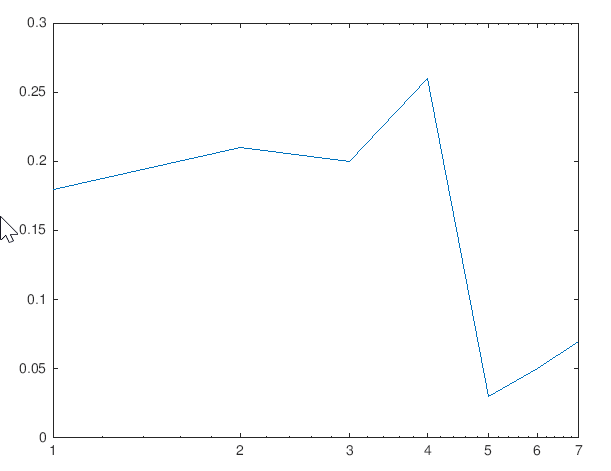Conclusion

It was safe to assume that wireless technology was one of the biggest technological asset to mankind. However, the human needs towards technologies changes with time and the need of a much more sophisticated and an efficient method was always closer to the horizon. Through the help of the technical report presented, the understanding towards prevailing wireless communication technologies are achieved.Numerous techniques had been thought about and had been applied for the achieving a simpler yet effective process of uplink into a wireless communication, and the research paper was thoroughly based onto these analyses. However, the allocation of any resource was precisely dependent onto the spectrum and the bandwidth allowed to a wireless communication.For the desired efficiency of the network system, a higher or a finitely equivalent spectral width had to be achieved. Through the findings of the research, it was observed that technologies which had been in use till date had many a flaws, thus, which was overcome with the help of a newer and a better technological replacement.

Dont miss your chance to excel in ME502 Overview of Digital Communication Assignment Help service! Hire tutor of Expertsminds.com for perfectly written assignment solutions!

Tag This :- Save top grade using ME502 Overview of Digital Communication Assignment Help service of Expertsminds.com.### Assignment Samples

 BSBHRM513 Manage Workforce Planning Assignment Help bsbhrm513 manage workforce planning assignment help - BSB51918 Diploma of Leadership and Management - analyse organisational data relating to staff turnover. "Imagery", in the assigned poem "The Fish" Assignment Help "imagery", in the assigned poem "the fish" assignment help - The use of imagery , symbolism all have been described & the concept of the poem has been described Organizational Diversity Assessment Help organizational diversity assessment help - This study is based on the case study analysis, effects and needs for diversity management in workplaces Human Resource Management (HRM) Assignment Help The focus is on how the UBER and Apple company are being in conflict with the problem of the ego. It includes the discussion of the different forms. Policies for the Prevention of Elder Abuse Assignment Help the research is about serving the different agendas and planning about the direct fashion which will help in addressing the decisions. Choice Of HEI In Foreign Countries Assignment Help choice of hei in foreign countries assignment help - In your critique, identify the main themes in the literature that are relevant to the purpose of the report Secured Design of Wireless LAN Assignment Help secured design of wireless lan assignment help - Melbourne Institute of Technology, Australia, Design and implementation of wireless LAN for a small campusGet Academic Excellence with Best Skilled Tutor! Order Assignment Now!